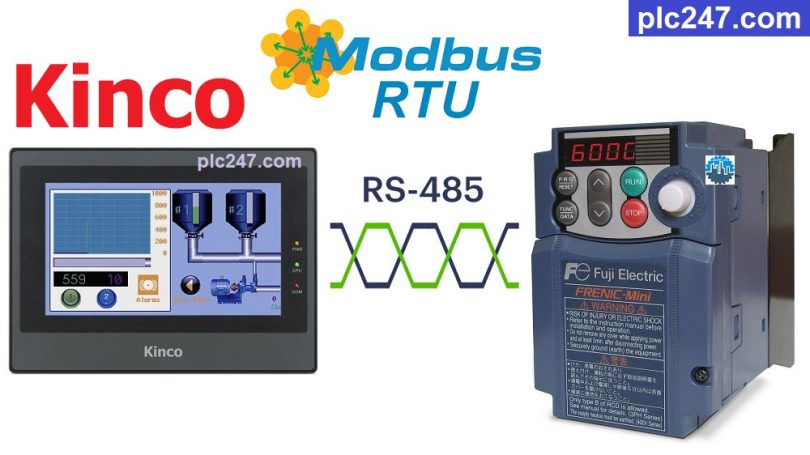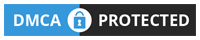# HMI Kinco “Modbus RTU” FUJI Frenic VFD TutorialWritten by

Fuji Frenic series inverters have energy-saving functions, simple operation, suitable for a wide range of applications with equipment operating from 0.1-15kW. And it also has RS485 communication support, convenient for communication with Modbus RTU protocol.

Kinco HMI is a cheap but good quality HMI, fully supporting the basic connections required of HMIs such as: RS232, RS422/RS485, Ethernet… Today plc247.com will guide the you use the Kinco HMI to control the Fuji Frenic inverter via Modbus RTU.

#### Kinco MT4414TE & Fuji Frenic “Wiring Diagram”

+ Most of the COM.0 ports of all Kinco HMIs are RS485-2 wires, and in this project I use this port to connect to the Fuji Frenic inverter.

#### Fuji Frenic “Modbus RTU” Parameters Setting

In addition to the basic parameters of the inverter installed according to the motor (frequency, current, voltage …) then to be able to control and monitor the inverter by Modbus RTU we need to install additional parameters following:

+ H30: 3 (VFD Control via RS485 Port)

+ Y02: 0

+ Y03: 2

+ Y04: 2 (9600bps)

+ Y05: 0 (Data: 8bit)

+ Y06: 1 (Parity Check: Even)

+ Y07: 1 (Stop Bit: 1)

+ Y10: 0 (Modbus-RTU Selection)

+ Y99: 0

##### Fuji Frenic Inverter Group Address Mapping
• Functions Mapping
##### Note: HMI Kinco Holding Registers Address = “VFD Address (Dec) + 1“

>>> Frequency reference = S(07H) + 05 = 705 (hexa) = 1797 (dec)
+ Frequency Setting Address = 1797 + 1 = 1798 (dec)

>>> Operation command = S(07H) + 06 = 706 (hexa) = 1798 (dec)
>>> Motor Control Address = 1798 + 1 = 1799 (dec)

• Set Word “1799” = 1 >>> Forward Run
= 2 >>> Reverse Run
= 0 >>> Stop Run

>>> Output frequency = M(08H) + 09 = 809 (hexa) = 2057 (dec)
+ Frequency Output Address = 2057 + 1 = 2058 (dec)

>>> Output current = M(08H) + 11 = 811 (hexa) = 2059 (dec)
+ Current Output Address = 2059 + 1 = 2060 (dec)

>>> Output voltage = M(08H) + 12 = 812 (hexa) = 2060 (dec)
+ Current Output Address = 2060 + 1 = 2061 (dec)

#### Kinco HMI “Modbus RTU” Configuration

How did you set the communication parameters for the Fuji Frenic inverter, the same settings for the HMI Kinco MT4414TE

### HMI Programming

+ Control Panel Design

+ Frequency Setting

+ “Run Forward” Command

+ “Run Reverse” Command

+ “Stop” Command

### Project Video Tutorial

======

###### Refereces